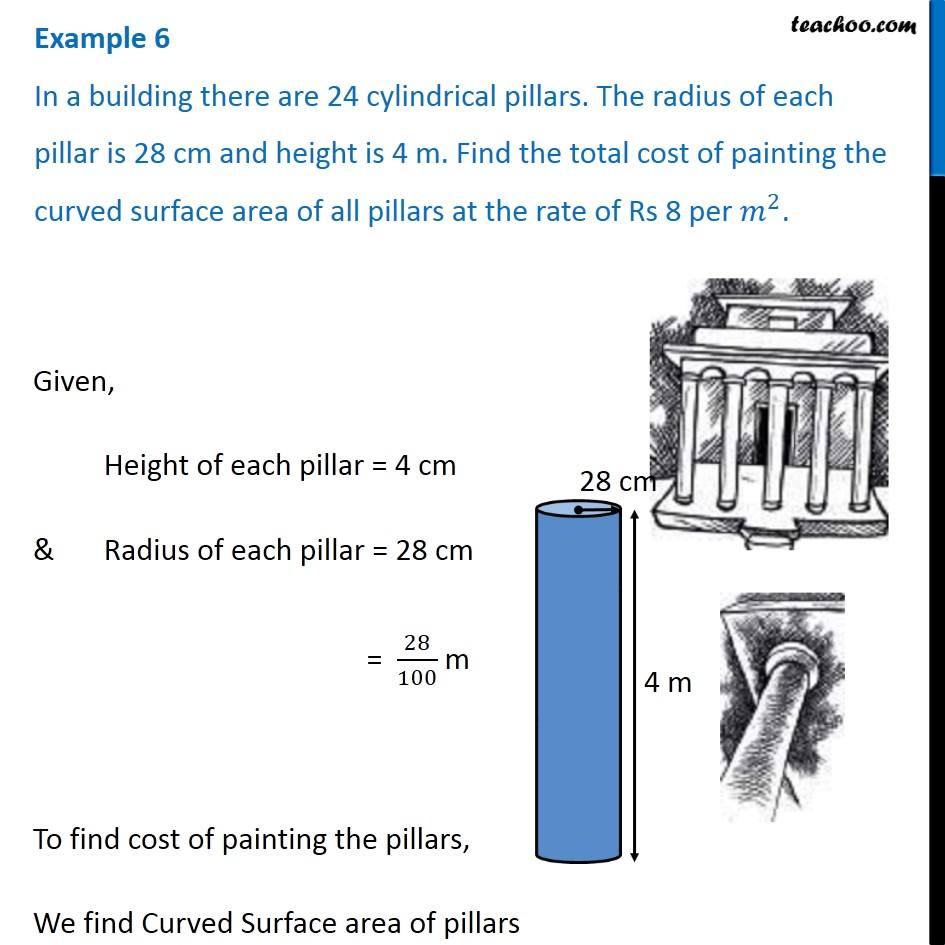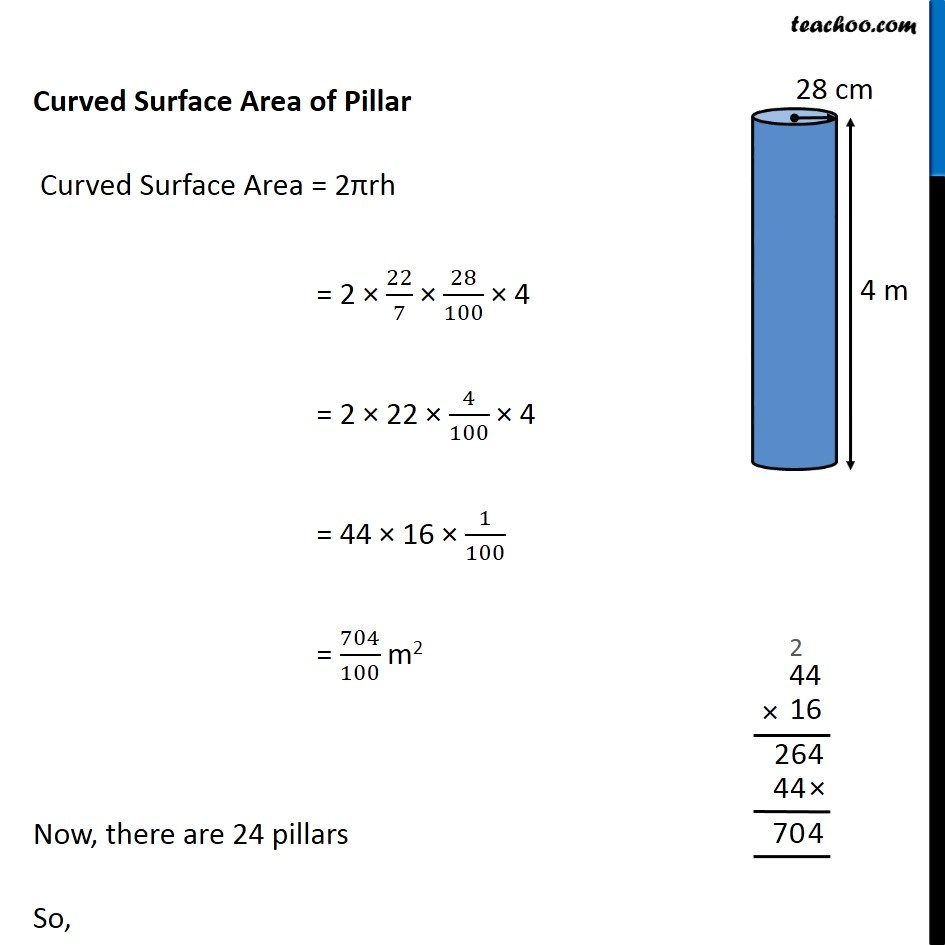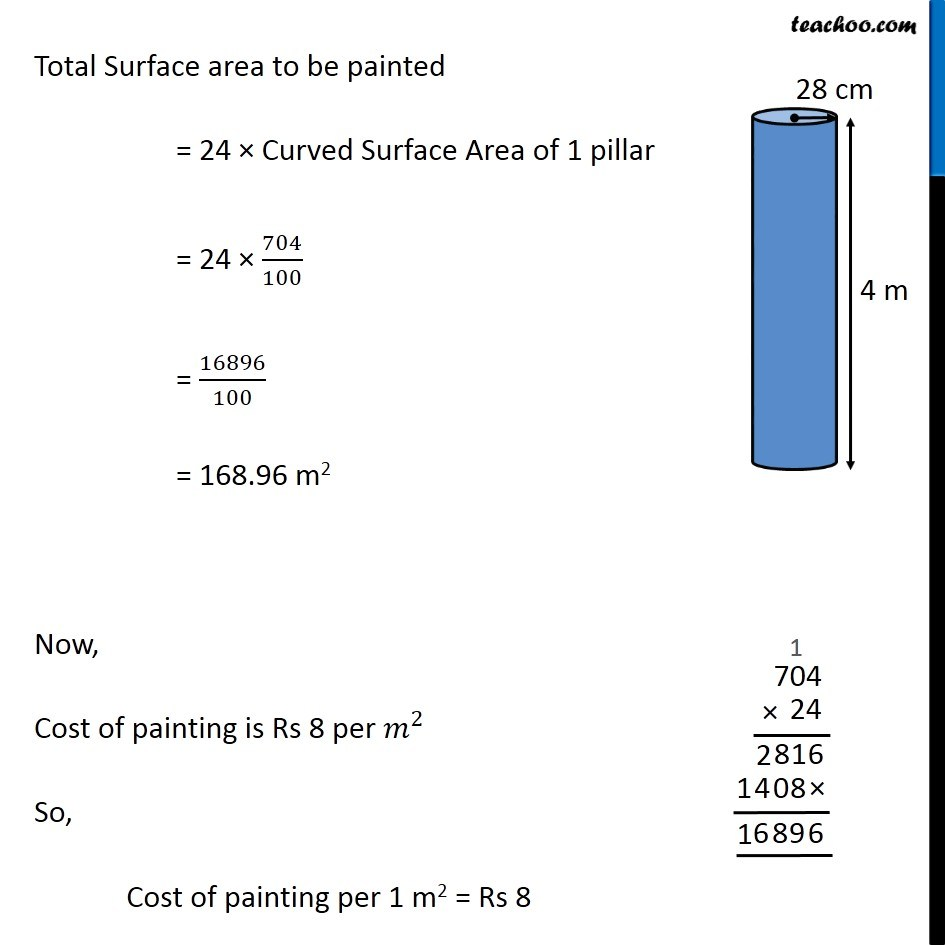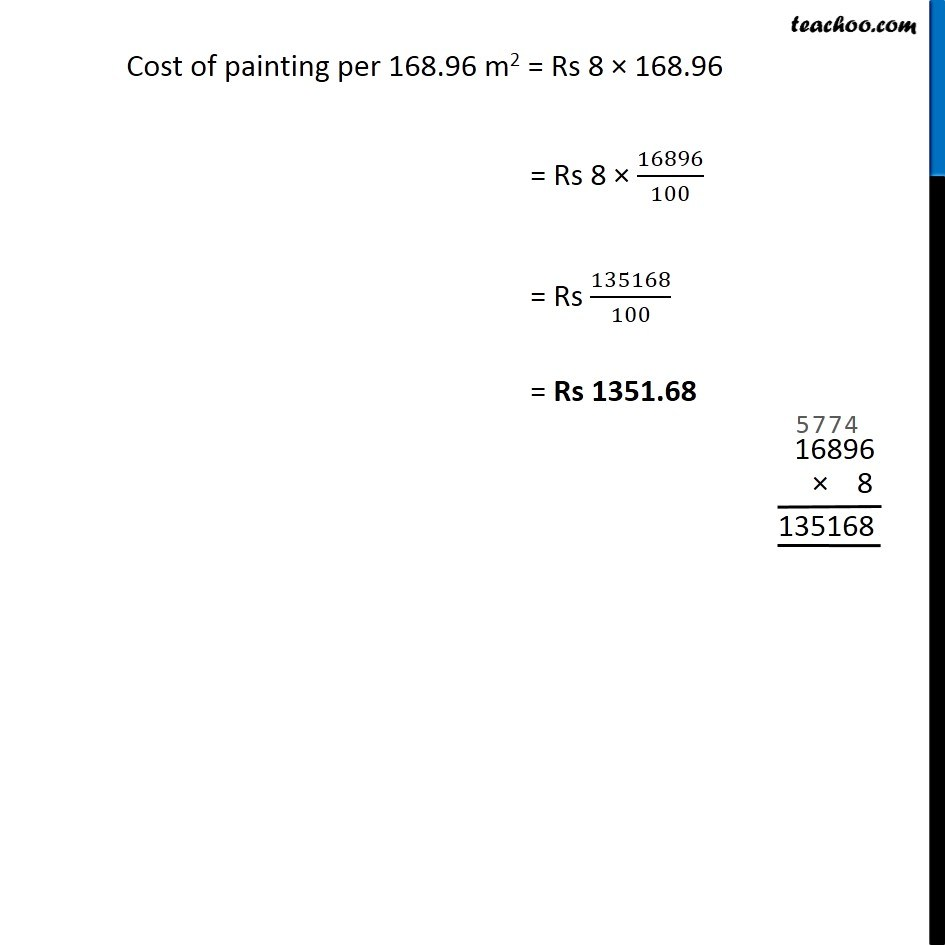Examples

Chapter 9 Class 8 Mensuration
Serial order wiseLearn in your speed, with individual attention - Teachoo Maths 1-on-1 Class

### Transcript

Example 6 In a building there are 24 cylindrical pillars. The radius of each pillar is 28 cm and height is 4 m. Find the total cost of painting the curved surface area of all pillars at the rate of Rs 8 per〖 𝑚〗^2. Given, Height of each pillar = 4 cm & Radius of each pillar = 28 cm = 28/100 m To find cost of painting the pillars, We find Curved Surface area of pillars Curved Surface Area of Pillar Curved Surface Area = 2πrh = 2 × 22/7 × 28/100 × 4 = 2 × 22 × 4/100 × 4 = 44 × 16 × 1/100 = 704/100 m2 Now, there are 24 pillars So, Total Surface area to be painted = 24 × Curved Surface Area of 1 pillar Total Surface area to be painted = 24 × Curved Surface Area of 1 pillar = 24 × 704/100 = 16896/100 = 168.96 m2 Now, Cost of painting is Rs 8 per〖 𝑚〗^2 So, Cost of painting per 1 m2 = Rs 8 Cost of painting per 168.96 m2 = Rs 8 × 168.96 = Rs 8 × 16896/100 = Rs 135168/100 = Rs 1351.68